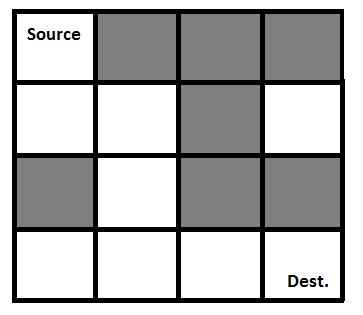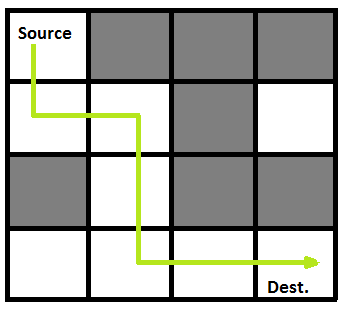GFG App
Open AppBrowser
Continue

# Java Program for Rat in a Maze | Backtracking-2

We have discussed Backtracking and Knight’s tour problem in Set 1. Let us discuss Rat in a Maze as another example problem that can be solved using Backtracking.

A Maze is given as N*N binary matrix of blocks where source block is the upper left most block i.e., maze, and destination block is lower rightmost block i.e., maze[N-1][N-1]. A rat starts from the source and has to reach its destination. The rat can move only in two directions: forward and down.

In the maze matrix, 0 means the block is a dead end, and 1 means the block can be used in the path from source to destination. Note that this is a simple version of the typical Maze problem. For example, a more complex version can be that the rat can move in 4 directions and a more complex version can be with a limited number of moves.

Following is an example maze.

` Gray blocks are dead ends (value = 0). `Following is binary matrix representation of the above maze.

```                {1, 0, 0, 0}
{1, 1, 0, 1}
{0, 1, 0, 0}
{1, 1, 1, 1}```

Following is a maze with highlighted solution path.Following is the solution matrix (output of program) for the above input matrix.

```                {1, 0, 0, 0}
{1, 1, 0, 0}
{0, 1, 0, 0}
{0, 1, 1, 1}
All entries in solution path are marked as 1.```

## Java

 `/* Java program to solve Rat in a Maze problem using` `   ``backtracking */`   `public` `class` `RatMaze {` `    ``final` `int` `N = ``4``;`   `    ``/* A utility function to print solution matrix` `       ``sol[N][N] */` `    ``void` `printSolution(``int` `sol[][])` `    ``{` `        ``for` `(``int` `i = ``0``; i < N; i++) {` `            ``for` `(``int` `j = ``0``; j < N; j++)` `                ``System.out.print(``" "` `+ sol[i][j] + ``" "``);` `            ``System.out.println();` `        ``}` `    ``}`   `    ``/* A utility function to check if x, y is valid` `        ``index for N*N maze */` `    ``boolean` `isSafe(``int` `maze[][], ``int` `x, ``int` `y)` `    ``{` `        ``// if (x, y outside maze) return false` `        ``return` `(x >= ``0` `&& x < N && y >= ``0` `&& y < N && maze[x][y] == ``1``);` `    ``}`   `    ``/* This function solves the Maze problem using` `       ``Backtracking. It mainly uses solveMazeUtil()` `       ``to solve the problem. It returns false if no` `       ``path is possible, otherwise return true and` `       ``prints the path in the form of 1s. Please note` `       ``that there may be more than one solutions, this` `       ``function prints one of the feasible solutions.*/` `    ``boolean` `solveMaze(``int` `maze[][])` `    ``{` `        ``int` `sol[][] = { { ``0``, ``0``, ``0``, ``0` `},` `                        ``{ ``0``, ``0``, ``0``, ``0` `},` `                        ``{ ``0``, ``0``, ``0``, ``0` `},` `                        ``{ ``0``, ``0``, ``0``, ``0` `} };`   `        ``if` `(solveMazeUtil(maze, ``0``, ``0``, sol) == ``false``) {` `            ``System.out.print(``"Solution doesn't exist"``);` `            ``return` `false``;` `        ``}`   `        ``printSolution(sol);` `        ``return` `true``;` `    ``}`   `    ``/* A recursive utility function to solve Maze` `       ``problem */` `    ``boolean` `solveMazeUtil(``int` `maze[][], ``int` `x, ``int` `y,` `                          ``int` `sol[][])` `    ``{` `        ``// if (x, y is goal) return true` `        ``if` `(x == N - ``1` `&& y == N - ``1``) {` `            ``sol[x][y] = ``1``;` `            ``return` `true``;` `        ``}`   `        ``// Check if maze[x][y] is valid` `        ``if` `(isSafe(maze, x, y) == ``true``) {` `            ``// mark x, y as part of solution path` `            ``sol[x][y] = ``1``;`   `            ``/* Move forward in x direction */` `            ``if` `(solveMazeUtil(maze, x + ``1``, y, sol))` `                ``return` `true``;`   `            ``/* If moving in x direction doesn't give` `               ``solution then  Move down in y direction */` `            ``if` `(solveMazeUtil(maze, x, y + ``1``, sol))` `                ``return` `true``;`   `            ``/* If none of the above movements works then` `               ``BACKTRACK: unmark x, y as part of solution` `               ``path */` `            ``sol[x][y] = ``0``;` `            ``return` `false``;` `        ``}`   `        ``return` `false``;` `    ``}`   `    ``public` `static` `void` `main(String args[])` `    ``{` `        ``RatMaze rat = ``new` `RatMaze();` `        ``int` `maze[][] = { { ``1``, ``0``, ``0``, ``0` `},` `                         ``{ ``1``, ``1``, ``0``, ``1` `},` `                         ``{ ``0``, ``1``, ``0``, ``0` `},` `                         ``{ ``1``, ``1``, ``1``, ``1` `} };` `        ``rat.solveMaze(maze);` `    ``}` `}` `// This code is contributed by Abhishek Shankhadhar`

Output:

```1  0  0  0
1  1  0  0
0  1  0  0
0  1  1  1```

Time Complexity: O(2^(n^2))

Auxiliary Space: O(n^2)

Please refer complete article on Rat in a Maze | Backtracking-2 for more details!

My Personal Notes arrow_drop_up